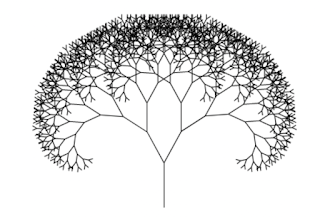## Saturday, 9 September 2023

### Practical causal ordering: Why weighted-Directed Acyclic Graphs (DAGs) are powerful for causal inference?

PreambleFractal Tree (Wikipedia)
A quiet causal inference revolution is underway in industry. We see immense success of transformers deep learning architectures. However their success should also be attributed to causal modelling. Large Language Models (LLMs), specially closed-sourced ones, elevates their performance with encoding causal mechanism with human designed deep learning components, i.e., innovative layers such as causal convolutions with multi-head self-attention layers. Now a classical approach,  one of the corner-stone of causality, is expressing modelling variates' causal relationships with  Directed Acyclic Graphs (DAGs). This lead to causal analysis and preserving ordering. In this short tutorial, we cover these without graph theoretic language for practical causal ordering.

Understanding weighted Directed Acyclic Graphs (wDAGs) as causal data structure

We first define what is a directions and weights. Providing a notational definition via tuples of objects.

Definition (wDAG): A weighted Directed Acyclic Graph (wDAG)  $\mathscr{G_{c}}$  is defined as set of ordered triplets of weights and connected random variables, such that, $k$th  triplet $(w_{k}, x_{i}, x_{j})$ where by $w_{k} \in \mathbb{R}$ is the weight, an effect size, between two variates that $x_{i}$ effects $x_{j}$. There are constraints :

(i) No cyclic effects can be defined, necessarily $x_{i}$ can not be equal to $x_{j}$.

(ii) If there is a definition,  $(w_{k}, x_{i}, x_{j})$ the reverse can't be defined, i.e.,  so that $(w_{k}, x_{j}, x_{i})$ does not exist.

(iii) No two causal effects sizes can't be exactly equal, $w_{k}$ can not be equal to $w_{l}$, from the same causal variable,  meaning no simultaneous events caused by the same random variable. This prevents ambiguity of ordering and random tie-breaks are unnatural.

This definition is practical and do not introduce any graph theory jargon. We left the sizes of indices as an exercise.

Inducing Causal Order via wDAGs

By the very definition of wDAGs, the power of this definition is one can construct causal ordering.

Definition (Causal Ordering from wDAG): Given $\mathscr{G_{c}}$, we can construct causal ordering among random variates $O(i)$ for $x_{i}$ using directionality and weights from  $\mathscr{G_{c}}$:

(i) if there exist a triplet  $(w_{k}, x_{i}, x_{j})$, then ordering $x_{j} \succ x_{i}$, implies $x_{j}$ occurred before $x_{j}$, or cause of $x_{i}$ was $x_{j}$

(ii) if there are two or more triplets having the same first variates, ordering is induces by the effect size $w_{k}$ among them.

To provide a simple example, let's say we formed  a wDAG, $\mathscr{G_{c}} = \{ (0.1, x_{1}, x_{2}),(0.2, x_{1}, x_{3}), (1.1, x_{2}, x_{4}) \}$ then the following causal ordering is established  $x_{1} \succ x_{3} \succ x_{2} \succ x_{4}$, note the ordering of $x_{3}$ that took precedence on $x_{2}$ due to its weight.

Why LLMs with causal ordering are so successful?

Probably not very well spelled property of LLMs are having causal layers with deep learning elevating their ability to capture causal ordering in natural language so well, not only sequence. This is still in infancy from research perspective as LLMs are biologically not plausible engineered software systems act as lossy knowledge compressors, lossy part usually identified as hallucination.

Conclusion

We introduce basic definition of wDAGs without heavy graph theory jargon and provide hints on why causal ordering with wDAGs has an immense contribution in constructing useful LLMs.

• looper : Causality Resource List. [link]
• Pearl et. al. Causal Inference in Statistics [link]
• Shimizu et. al., A Linear Non-Gaussian Acyclic Model for Causal Discovery [link]
• Hamad, et. al, Dilated causal convolution with multi-head self attention for sensor human activity recognition [link].
• Lui et. al DecBERT: Enhancing the Language Understanding of BERT with Causal Attention Masks [link]